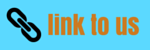# Class 9 Maths Worksheet for Number System

Notes Ncert Solutions Assignments Videos Revision sheet

Here we are trying to give the Class 9 Maths Worksheet for Number System. It tests the basic concepts and at the same makes the student comfortable with the questions

Question 1
Find the value of each of the Following
a) 161/4
b) 625-3/4
c) 2√3   + 8√18
d) 15√6 + √216
e) 105/2 / 103/2
f) 53/2  73/2
g) √162/√2
h) (1+√2)/(1-√2)
Question 2
Simplify the below expression
a)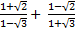b)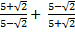c)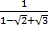d)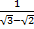Question 3
Find the value of a and b such that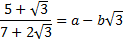Question 4
If p = 1+√3
Find the value of
i) p2 + 1/p2
ii) p4 + 1/p4
Question 5
State whether the following statements are true or false
(i) 2/√5    is a rational number.
(ii) There are infinitely many integers between any two integers.
(iii) Number of rational numbers between 11 and 13 is finite.
(iv) There are numbers which cannot be written in the form  p/q , q ≠ 0 , p, q both   are integers.
(v) The square of an irrational number is always rational.
(vi)  √18/√2   is not a rational number as √18 and √2 are not integers.
Solution
5)
i) False
ii) false
iii) false
iv) True
v) False
vi)  False